Linked to an optional connector or a Calculation node, the Parameter node defines the minimum and maximum values, and the number of decimal places for these values. The output value is set using a slider.

To add a Parameter node, right click in the Graph Editor and select Create Node  > Calculation  > Formula.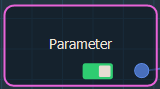Double click on the node to open its parameters: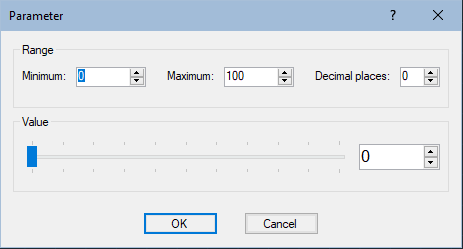Editing a Parameter node

The parameters window allows you to set the Range of the output value, and then you can use the slider to set an exact value.

• Enter the Minimum and Maximum values, number of decimal places for these values.
• Use the slider to set the output Value.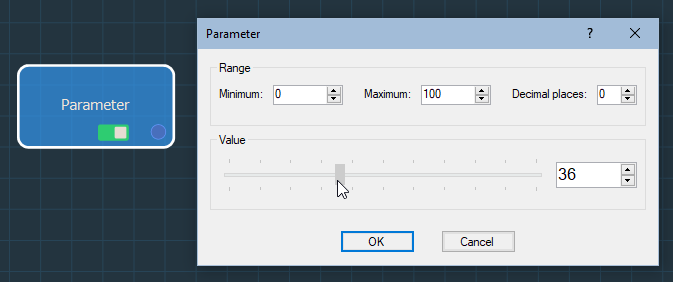The Parameter node has one output connector that links to a Calculation node or an optional connector.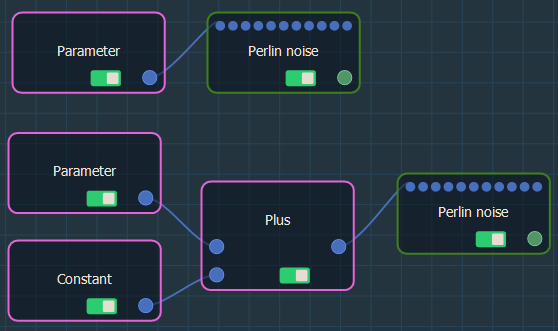Example

In the example below, we use two Parameter nodes to drive the terrain size of the Perlin noise generator and the maximum height of the Min & max heights terrain transformer.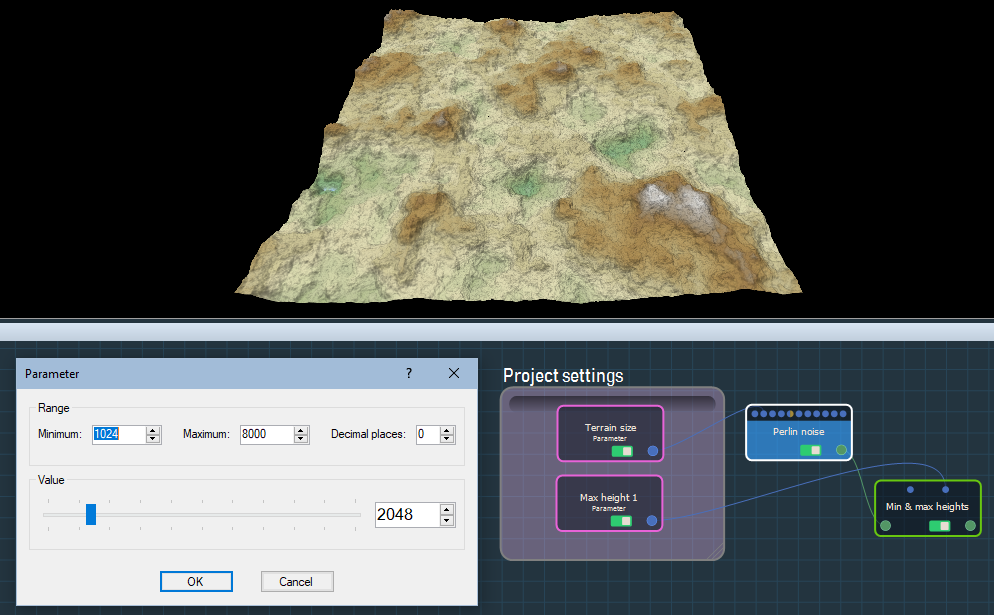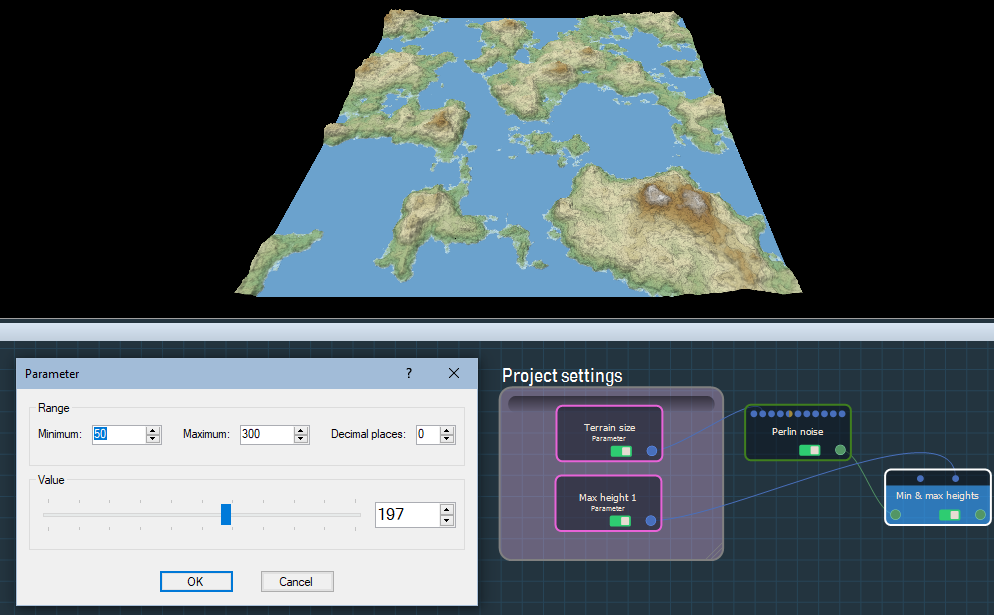Parameters

Parameter Use
Minimum Sets a minimum output value
Maximum Sets a maximum output value
Value Defines the exact output value